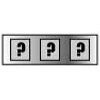#### You may also like### Summing Consecutive Numbers

15 = 7 + 8 and 10 = 1 + 2 + 3 + 4. Can you say which numbers can be expressed as the sum of two or more consecutive integers?### Forgotten Number

I have forgotten the number of the combination of the lock on my briefcase. I did have a method for remembering it...### Series Sums

Let S1 = 1 , S2 = 2 + 3, S3 = 4 + 5 + 6 ,........ Calculate S17.

# Take Ten Sticks

##### Age 11 to 16Challenge Level

This problem is good to encourage systematic thinking - working from a simple start: two sticks, three, four . . .

Encourage questions like "what could come before . . . . . and what before that" ?

Working backwards from the goal is a mathematical thinking skill.

Especially encourage explanation - accounting for pattern.

There is lots of interest in this simple mechanical process - look out for the triangle numbers!

Above all, if possible, let each insight prompt fresh questions to consider, each new question will illuminate more of the structure.